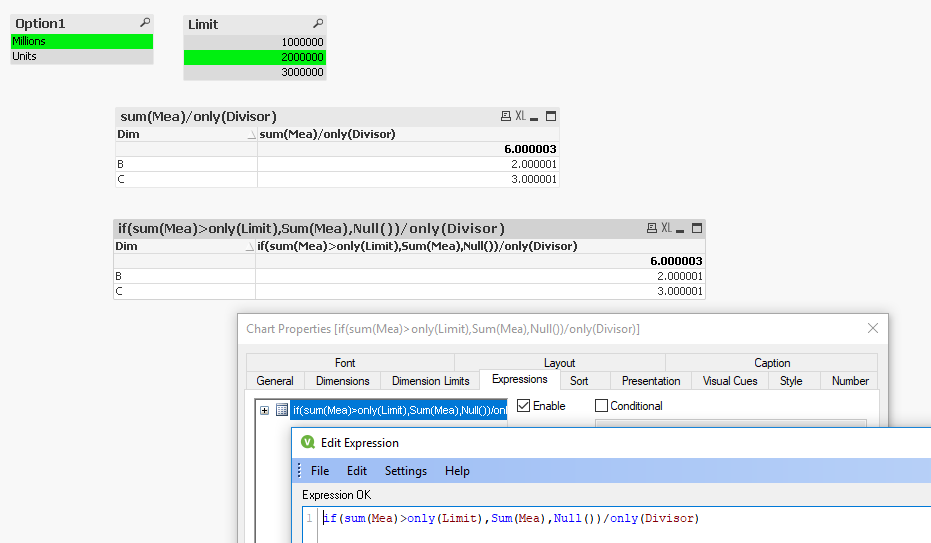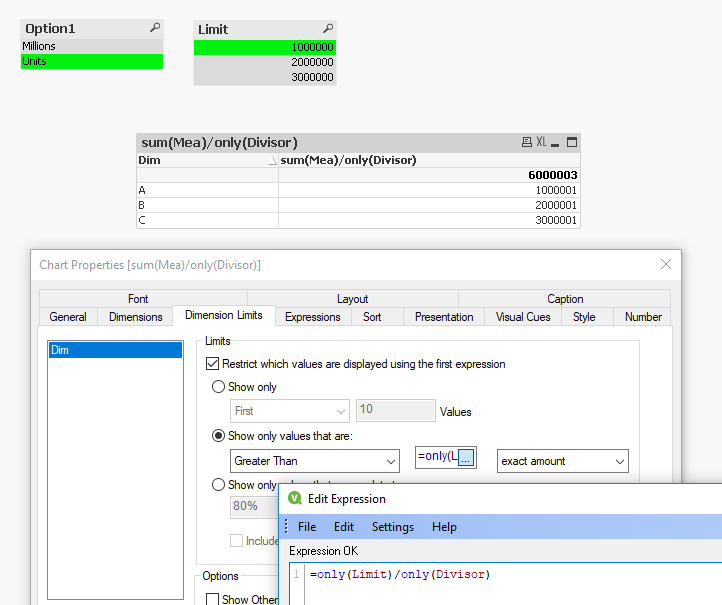# QlikView App Dev

Discussion Board for collaboration related to QlikView App Development.

Announcements
Join this live chat April 6, 10AM EST - QlikView to Qlik Sense REGISTER
cancel
Showing results for
Search instead for
Did you mean:Creator II

## ADD one more Option in Expression

Hi Team,

I have scenario, Like this.

I have 3 List box.

Option 1 - Million and Full, When User is selecting Million, Value is coming like 1.1 M, 1.45 M and When User is Selecting Full, Value is coming 1000000.45, 1010000 ...... in Straight Table

Option 2 - No and Yes, When User is selecting No, Some Value is adding and When user is Selecting Yes, Value is extracting in Straight Table

Both is working well.

Now I have to add one more option, i.e - Option 3 - 1M, 2M and 3M, Suppose User select the 1M, Now I want only greater than 1M value record in my straight table.

Similarly for 2 Million and 3 Million. But my option 1 and option 2 should also work with Option 3.

Below is my Expression :

IF(GetFieldSelections(Option1)='Millions', IF(GetFieldSelections(Option2)='No',

sum({<sub_account={'EXPENSE'}>}Amount)/1000000, (Sum({<sub_account={'EXPENSE'}>}Amount_Ex_CC - Amount_Ex))/1000000),

IF(GetFieldSelections(Option2)='No', sum({<sub_account={'EXPENSE'}>}Amount),(Sum({<sub_account={'EXPENSE'}>}Amount_Ex_CC - Amount_Ex))))

This is single expression.

Can anyone add new option 3 in my above expression.

Regards,

AK

Labels (2)

• ### Expression

1 Solution

Accepted SolutionsSpecialist

Hi,

So if you don't want to use the dimension limits but are happy to use the limit table per your post then your pattern involving the least repetition will be;

if(sum(Mea)>only(Limit),Sum(Mea),Null())/only(Divisor)

Slotting in your functions I think would give;

if(if(GetFieldSelections(Option2)='No', Sum({<account={'EXPENSE'}>}Amount), Sum({<sub_account={'EXPENSE_TYPE'}>}Amount_CC))>only(Num),if(GetFieldSelections(Option2)='No', Sum({<account={'EXPENSE'}>}Amount), Sum({<sub_account={'EXPENSE_TYPE'}>}Amount_CC)),Null())/if (GetFieldSelections(Option1)='Millions',1000000,1)

Which will only work when you have something selected on Option table.

Cheers,

Chris.7 RepliesSpecialist

Hi,

I would take a different approach to simplify your expressions.

From the toy script at the bottom you get a disconnected table that lets you use Only(Divisor) to divide as your Option1 replacement. You would just put that at the end. Strength here is when someone says can you also do thousands … you just add to the table.

To impose the limit you could again have a Limit table disconnected (or maybe even just a variable) & use dimension limits.

You still use your IF statement for the other option, but would hopefully be easier to follow.Cheers,

Chris.

``````divisor:
LOAD * INLINE [
Option1, Divisor
Millions, 1000000
Units, 1
];

limit:
LOAD * INLINE [
Limit
1000000
2000000
3000000
];

data:
LOAD * INLINE [
Dim, Mea
A, 1000001
B, 2000001
C, 3000001
];``````Creator II
Author

Hi Chrismarlow,

Is it not possible to make any expression which I have written for Option1 and Option2. So If I am using Option 3, How I can put that condition in my expression.

IF(GetFieldSelections(Option1)='Millions', IF(GetFieldSelections(Option2)='No',

sum({<sub_account={'EXPENSE'}>}Amount)/1000000, (Sum({<sub_account={'EXPENSE'}>}Amount_Ex_CC - Amount_Ex))/1000000),

IF(GetFieldSelections(Option2)='No', sum({<sub_account={'EXPENSE'}>}Amount),(Sum({<sub_account={'EXPENSE'}>}Amount_Ex_CC - Amount_Ex))))

Regards,

AKCreator II
Author

Hi Chrismalow

Is it possible by expression, Below is required information:

I have a listbox : 1M, 2M and 3M, Created by Inline function, Below is script :

Option:
Load Inline [
Option, Num
'1 M \$', 1000000
'2 M \$', 2000000
'3 M \$', 3000000
];

I have a straight table, Where All the possible value is displaying.

Now I want when I select 1M\$, only greater than 1M value should to display in Straight Table.

When I select 2M\$, only greater than 2M value should to display in Straight Table, Similarly to 3M.

How I can do the same: Below is my Expression.

If (GetFieldSelections(Option1)='Millions', if(GetFieldSelections(Option2)='No', Sum({<account={'EXPENSE'}>}Amount)/1000000, Sum({<sub_account={'EXPENSE_TYPE'}>}Amount_CC)/1000000),
if(GetFieldSelections(Option2)='No', Sum({<sub_account={'EXPENSE'}>}Amount), Sum({<sub_account={'EXPENSE'}>}Amount_CC))))

Can you feed the logic for the same.

Thanks,

AKCreator II
Author

Is there any solution for the same.

Regards,

AKSpecialist

Hi,

So if you don't want to use the dimension limits but are happy to use the limit table per your post then your pattern involving the least repetition will be;

if(sum(Mea)>only(Limit),Sum(Mea),Null())/only(Divisor)

Slotting in your functions I think would give;

if(if(GetFieldSelections(Option2)='No', Sum({<account={'EXPENSE'}>}Amount), Sum({<sub_account={'EXPENSE_TYPE'}>}Amount_CC))>only(Num),if(GetFieldSelections(Option2)='No', Sum({<account={'EXPENSE'}>}Amount), Sum({<sub_account={'EXPENSE_TYPE'}>}Amount_CC)),Null())/if (GetFieldSelections(Option1)='Millions',1000000,1)

Which will only work when you have something selected on Option table.

Cheers,

Chris.Creator II
Author

Lots of thanks. 😊Specialist

You are welcome.